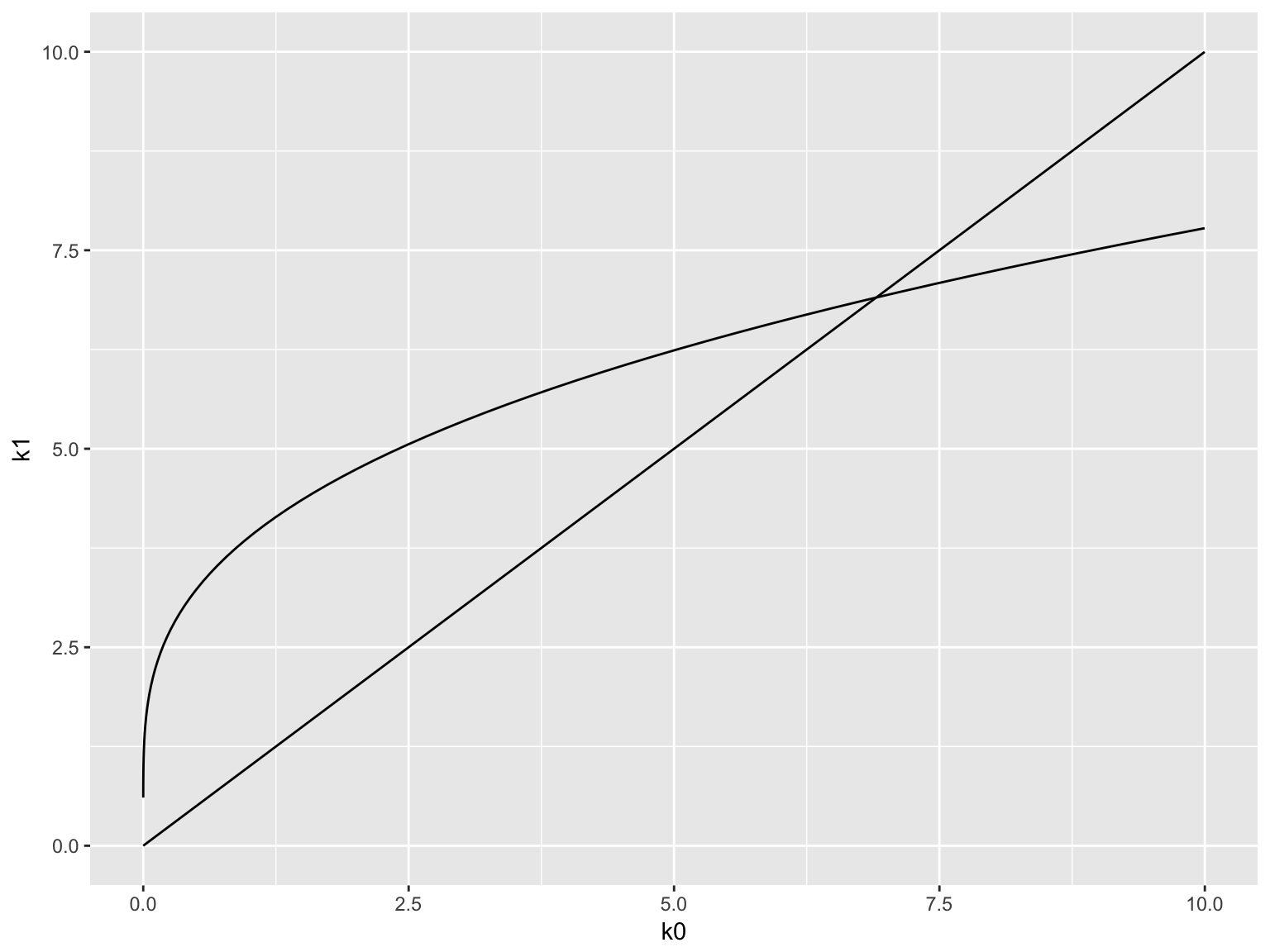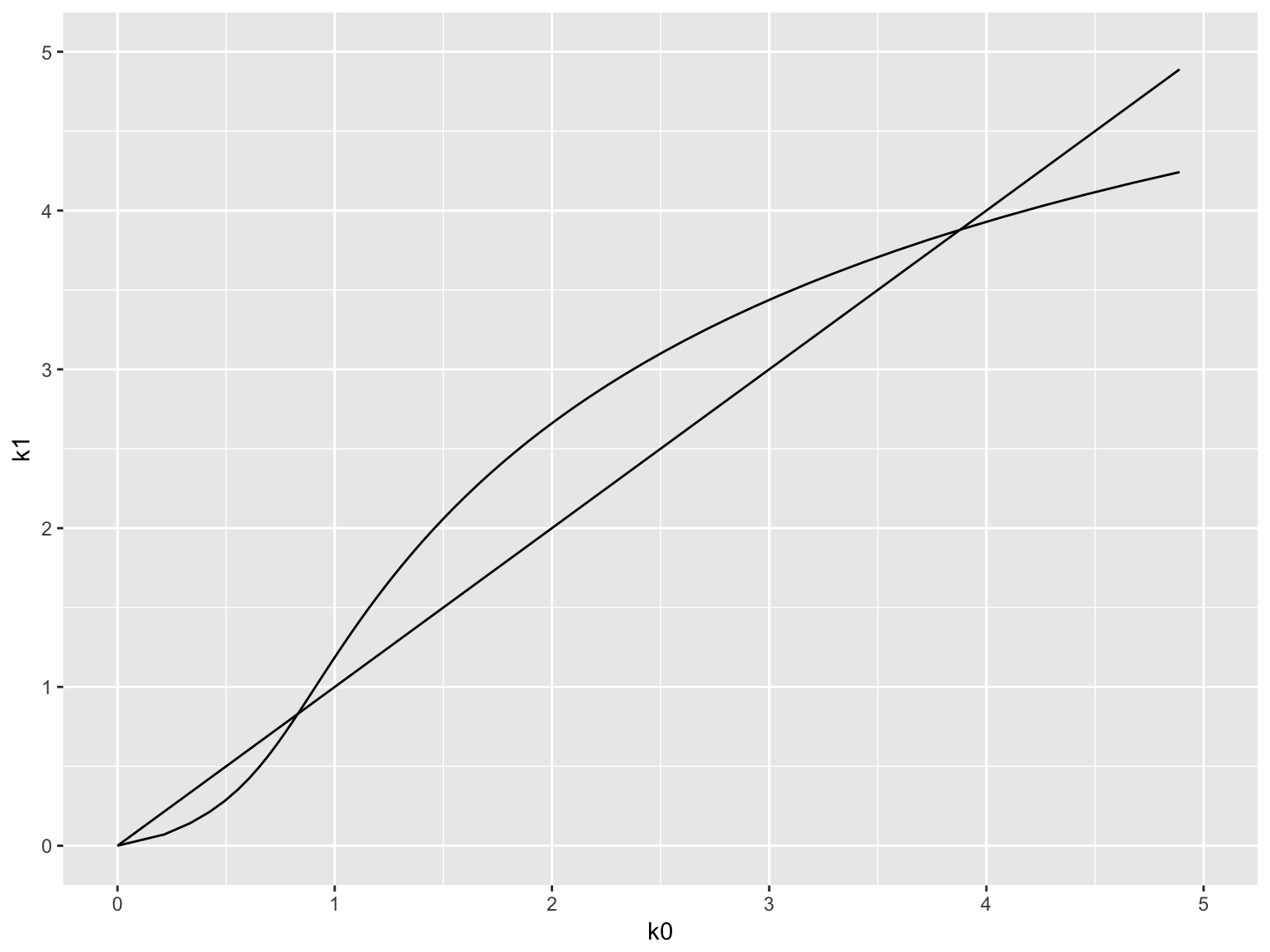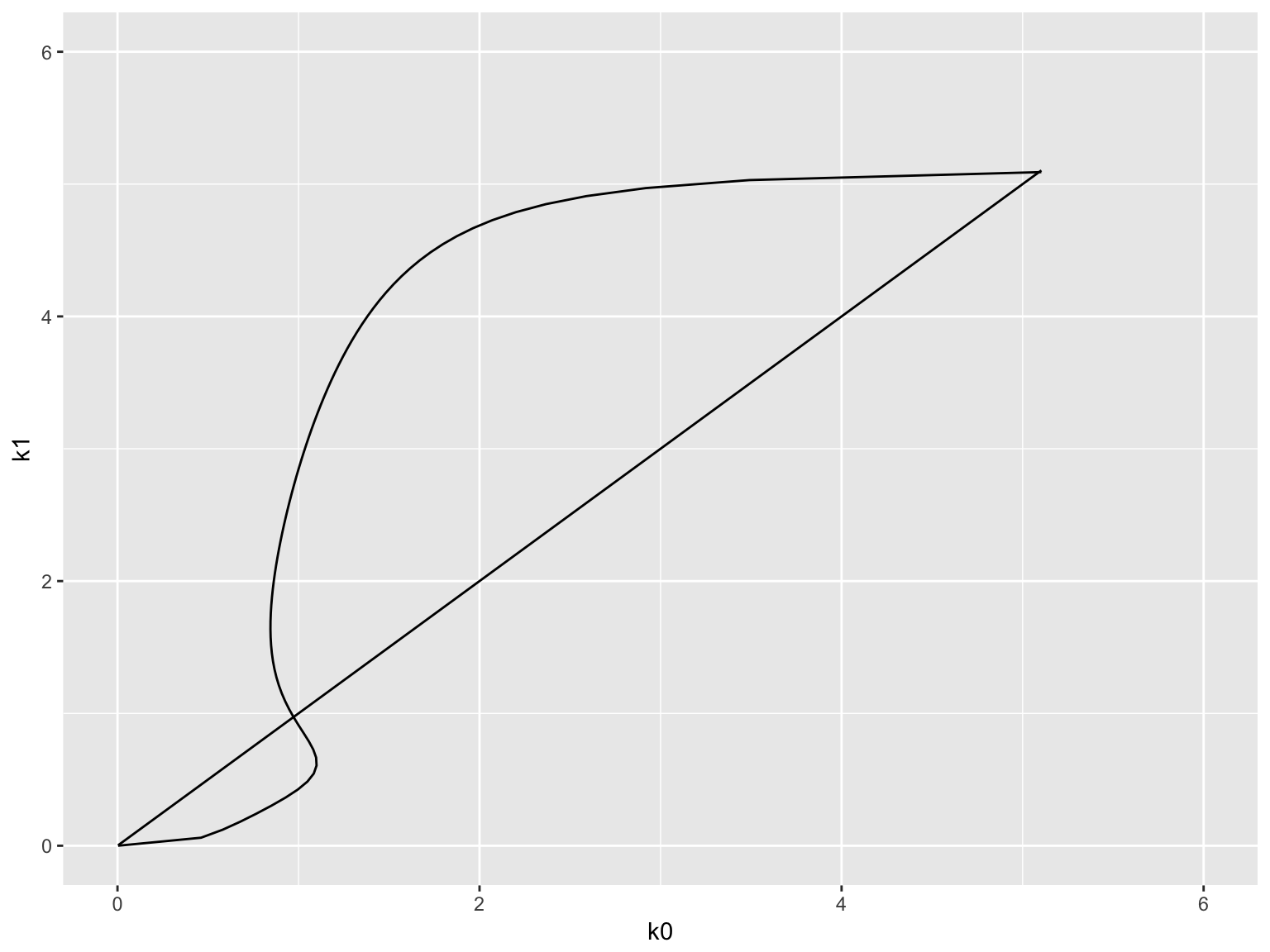January 27, 2017

# Dynamics of the OLG model

The dynamics of the capital per unit of effective labor $$\hat k_t$$ obeys

$\hat{k}_{t+1} = \frac{s\left(f'(\hat{k}_{t+1})\right) \left[f(\hat{k}_{t})-\hat{k}_{t}f'(\hat{k}_{t})\right]} {(1+g)(1+n)},$

This is an implicit system (the left- and right-hand sides both contain $$\hat{k}_{t+1}$$).

$$k_{t+1}$$ may not be a function of $$k_{t}$$ in which case, there is multiple possibilities for the time path.

# Cobb-Douglas Production and Log Utility

To obtain a clear result, we assume that

$f(k)=k^{\alpha},\quad0<\alpha<1$

and the log instantaneous utility function: i.e., $$\theta=1$$.

Prove that the dynamics is characterized by

$\hat{k}_{t+1}=\frac{(1-\alpha)}{(1+g)(1+n)(2+\rho)}\hat{k}_{t}^{\alpha}$

Looks like the Solow model.

# Cobb-Douglas Production and Log Utility (Stability)

It can be shown that for any $$\hat k_0 > 0$$

$\hat k_t \to \hat{k}^{*}=\left[\frac{1-\alpha}{(1+n)(1+g)(2+\rho)}\right]^{\frac{1}{1-\alpha}}$

as $$t \to \infty$$.

The prediction of the Solow model is preserved. For instance, per-capita capital

$k_t = A_t \hat k_t$

grows at the rate of $$g$$ in the long run.

# Dynamic Inefficiency

OLG models may have dynamic inefficiency in that at least one generation can increase utility without nobody else reducing utility.

Let’s continue to assume that the production function is C-D and utility is log. The steady state for capital per unit of effective labor satisfies

$\hat{k}^{*}=\left[\frac{1-\alpha}{(1+n)(1+g)(2+\rho)}\right]^{\frac{1}{1-\alpha}}$

\begin{aligned} f'\left(\hat{k}^{*}\right) &= \alpha\left(\left[ \frac{1-\alpha}{(1+n)(1+g)(2+\rho)} \right]^{\frac{1}{1-\alpha}}\right)^{\alpha-1}\\ &= \frac{\alpha(1+n)(1+g)(2+\rho)}{1-\alpha}. \end{aligned}

# Dynamic Inefficiency (cont’d)

The golden rule capital stock $$\hat k_G$$ satisfies (under $$\delta = 0$$):

$f'(\hat{k}_{G}) = n + g$

Thus,

$\frac{\alpha(1+n)(1+g)(2+\rho)}{1-\alpha} < n + g \Longleftrightarrow \hat{k}^* > \hat k_G$

If $$\alpha$$ is sufficiently small, the above inequalities hold and it gives rise to dynamic inefficiency.

In OLG models, the fact that there is no coordination between generations may result in too much saving.

# Reallocation

Let’s suppose $$\hat{k}^* > \hat k_G$$ and that the economy is on the BGP.

If some social planner forces

• young people in period $$t_{0}$$ to consume more and save less by some amount $$\Delta > 0$$ by which the economy moves to $$\hat{k}_{G}$$,
• people in subsequent periods consume the golden rule level

Consumption per effective worker in period $$t_{0}$$ satisfies:

\begin{aligned} f(\hat{k}^{*})+(\hat{k}^{*}-\hat{k}_{G})-(g+n)\hat{k}_{G} &> f(\hat{k}_{G})-(g+n) \hat{k}_{G}\\ &> f(\hat{k}^{*})-(g+n)\hat{k}^{*}. \end{aligned}

# Reallocation (cont’d)

$f(\hat{k}^{*})+(\hat{k}^{*}-\hat{k}_{G})-(g+n)\hat{k}_{G}$

-> consumption in period $$t_0$$

$f(\hat{k}_{G})-(g+n) \hat{k}_{G}$

-> consumption in period $$t > t_0$$

$f(\hat{k}^{*})-(g+n)\hat{k}^{*}$ -> consumption without the reallocation.

All generations potentially become better off by this reallocation. The original saving rate was too high.

# Dynamic efficiency of the Ramsey model

In the Ramsey model, it always holds that

$\hat k^* < \hat k_G$

So, there is no dynamic inefficiency in the Ramsey model.

# Multiple equilibrium

Let’s go back to the implicit equation. By rearranging terms, we have

$f(\hat{k}_{t})-\hat{k}_{t}f'(\hat{k}_{t}) = \frac{(1+g)(1+n)\hat k_{t+1}}{s\left( f'(\hat k_{t+1}) \right)}$

Define $$w(k) = f(k) - kf'(k)$$. Since

• $$w(k)$$ is increasing in $$k$$
• $$w(0) = 0$$, and
• the right-hand side is positive

Given $$\hat k_{t+1}$$, we can solve for a unique $$\hat k_t$$ that satisfies the above equation. We have the inverse dynamics ($$\hat k_{t+1} \mapsto \hat k_t$$)

# Code

OLG = function(theta, rho, g, n, alpha, gamma, A) {
f = function(k) A * (alpha * k ^ gamma + 1 - alpha) ^ (1 / gamma)
df = function(k) A * alpha * (alpha + (1 - alpha) * k ^ (-gamma)) ^
((1 - gamma) / gamma)
w = function(k) f(k) - k * df(k)
w_inv = function(y) {
sol = nleqslv::nleqslv(100, function(x) w(x) - y)
if (sol$termcd == 1) return(sol$x) else return(NA)
}
s = function(r){
sigma = (1 - theta) / theta
return((1 + r) ^ sigma) / ((1 + rho) ^ (1 / theta) + (1 + r) ^ sigma)
}
w2 = function(k) (1 + g) * (1 + n) * k / s(df(k))
return(list(f=f, df=df, w=w, w_inv=w_inv, w2=w2))
}

# Typical Case

model = OLG(theta=0.8, rho=0.4, g=0.2, n=0.2,
alpha=0.33, gamma=0.1, A=7)

k1 = seq(0.0, 10, length.out=100)
k0 = lapply(model$w2(k1), model$w_inv)
k0 = unlist(k0)
qplot(k0, k1, geom='line') + geom_line(data=tibble(x=k0, y=k0), aes(x,y)) +
xlim(0.0, 10) + ylim(0.0, 10)# Multiple Equilibrium (Poverty Trap)

model = OLG(theta=8, rho=0.4, g=0.3, n=0.2,
alpha=0.33, gamma=-0.7, A=7)

k1 = seq(0.0, 7, length.out=100)
k0 = lapply(model$w2(k1), model$w_inv)
k0 = unlist(k0)
qplot(k0, k1, geom='line') + geom_line(data=tibble(x=k0, y=k0), aes(x,y)) +
xlim(0.0, 5) + ylim(0.0, 5)# Multiple Steady States (Self-fulfilling prophecy)

model = OLG(theta=8, rho=0.4, g=0.3, n=0.2,
alpha=0.33, gamma=-2.9, A=7)

k1 = seq(0.00, 6, length.out=100)
k0 = lapply(model$w2(k1), model$w_inv)
k0 = unlist(k0)
ggplot(data=tibble(k0=k0, k1=k1)) + geom_path(aes(x=k0, y=k1)) +
geom_line(aes(k0,k0)) + xlim(0.0, 6) + ylim(0.0, 6)# Multiple Steady States (Self-fulfilling prophecy)

Because the agents have three possible $$\hat k_{t+1}$$. The path they choose is affected by non-fundamental factors (called sunspots).
If they believe the economy experiences boom, they choose the higer $$\hat k_{t+1}$$ and the economy converges to the higher steady state. Their belief has the power to make it true.